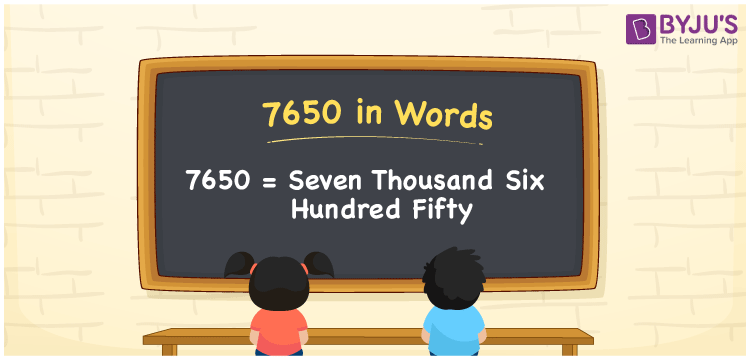# 7650 in words

7650 in words is written as Seven Thousand Six Hundred and Fifty. 7650 represents the count or value. The article on Counting Numbers can give you an idea about count or counting. The number 7650 is used in expressions that relate to money, distance, length, year and others. Let us consider an example for 7650. “Beena saved Seven Thousand Six Hundred and Fifty Rupees in a year.” Another example would be ”A free subscription had unlimited access for viewing content for Seven Thousand Six Hundred and Fifty minutes.”

 7650 in words Seven Thousand Six Hundred and Fifty Seven Thousand Six Hundred and Fifty in Numbers 7650

## 7650 in English Words## How to Write 7650 in Words?

We can convert 7650 to words using a place value chart. The number 7650 has 4 digits, so let’s make a chart that shows the place value up to 4 digits.

 Thousands Hundreds Tens Ones 7 6 5 0

Thus, we can write the expanded form as:

7 × Thousand + 6 × Hundred + 5 × Ten + 0 × One

= 7 × 1000 + 6 × 100 + 5 × 10 + 0 × 1

= 7650

= Seven Thousand Six Hundred and Fifty.

7650 is the natural number that is succeeded by 7649 and preceded by 7651.

7650 in words – Seven Thousand Six Hundred and Fifty.

Is 7650 an odd number? – No.

Is 7650 an even number? – Yes.

Is 7650 a perfect square number? – No.

Is 7650 a perfect cube number? – No.

Is 7650 a prime number? – No

Is 7650 a composite number? – Yes

## Solved Example

1. Write the number 7650 in expanded form

Solution: 7 × 1000 + 6 × 100 + 5 × 10 + 0 × 1

We can write 7650 = 7000 + 600 + 50 + 0

= 7 × 1000 + 6 × 100 + 5 × 10 + 0 × 1

## Frequently Asked Questions on 7650 in words

Q1

### How to write 7650 in words?

7650 in words is written as Seven Thousand Six Hundred and Fifty.
Q2

### State True or False. 7650 is divisible by 5?

True. 7650 is divisible by 5.
Q3

### Is 7650 a perfect square number?

No. 7650 is not a perfect square number.